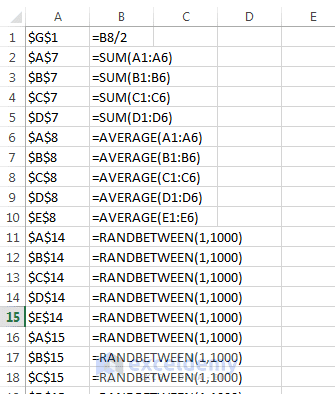Disclosure: This post may contain affiliate links, meaning when you click the links and make a purchase, we receive a commission.

# A Macro example created using VBA

The following is a VBA macro that you can’t create by recording. It uses programming concepts that must be entered manually. This macro shows a list of all formulas on the active sheet. The list will be created on a new worksheet.

```Sub ListFormulas()
'Create a range object
Set InputRange = ActiveSheet.UsedRange
'Variable for the output row
OutputRow = 1
'Loop through the range
For Each cell In InputRange
If cell.HasFormula Then
OutputSheet.Cells(OutputRow, 1) = "'" & cell.Address
OutputSheet.Cells(OutputRow, 2) = "'" & cell.Formula
OutputRow = OutputRow + 1
End If
Next cell
End Sub
```

This macro may look complicated to you at the first time, but this is really simple when you will break it down. Here’s how this code snippet works:

1. Sub ListFormulas(): This statement starts the macro subprocedure.
2. ‘Create a range object: A comment that describes the next statement Set InputRange = ActiveSheet.UsedRange.
3. Set InputRange = ActiveSheet.UsedRange: This statement creates an object variable that corresponds to the used range on the active sheet.
4. ‘Add a new sheet: This comment describes the next statement: Set OutputSheet = Worksheets.Add.
5. Set OutputSheet = Worksheets.Add: This statement adds a new worksheet and creates an object variable name OutputSheet.
6. ‘Variable for the output row: This is a comment for this statement: OutputRow = 1
7. OutputRow = 1: This statement sets 1 for the variable OutputRow.
8. ‘Loop through the range: A comment about the next For Each loop
9. For Each cell In InputRange: This is starting of For Each loop. The first cell is selected from the InputRange object variable.
10. If cell.HasFormula Then: This If-Then statement is checking whether the selected cell (from the InputRange object variable) has any Formula. If any formula then executes the next three statements:
OutputSheet.Cells(OutputRow, 1) = “‘” & cell.Address
OutputSheet.Cells(OutputRow, 2) = “‘” & cell.Formula
OutputRow = OutputRow + 1
11. OutputSheet.Cells(OutputRow, 1) = “‘” & cell.Address: This statement put the absolute cell address with an apostrophe in the first cell of row 1 in the new worksheet opened by this statement: Set OutputSheet = Worksheets.Add.
12. OutputSheet.Cells(OutputRow, 2) = “‘” & cell.Formula: This statement put the cell’s formula with an apostrophe sign in the second cell of row 2 in the new worksheet opened by this statement: Set OutputSheet = Worksheets.Add.
13. OutputRow = OutputRow + 1: This statement increases the value of OutputRow by 1.
14. End If: End the If-Then construct.
15. Next cell: This statement will select the next cell of object variable InputRange and will execute the No. 10 operation again. This will continue until all the cells in InputRange are manipulated.
16. End Sub: This statement ends the macro subprocedure.

The following figure shows the result of running this macro — a handy list of all formulas in the worksheet.The ListFormulas() macro creates a list of all formulas in a worksheet.

This macro is okay, but it is not the perfect one. It doesn’t show any message when it faces any kind of error. For example, if the workbook is protected by its creator, running this macro will show error as this macro adds a new worksheet.